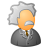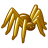# from_geo_coordinate_string

909
5
01-10-2018 08:24 AMby
New Contributor III

I have a WKT string in WGS 84:

```'LINESTRING (34.77491100000276 31.98871099999881,....
How do I load it into a geometry with the from_geo_coordinate_string function?```
5 RepliesbyMVP Esteemed Contributor

After reading the documentation on the function, arcgis.geometry module — arcgis 1.3.0 documentation , and trying it; what exactly are the errors or unexpected results you are getting?by
New Contributor III

It is not clear:

1. What is the conversion type - I do not need any as the data should stay at WGS84

2. Is a single string good? the documentation talks about an array

Here is the line of code:

l = 'LINESTRING (34.77491100000276 31.98871099999881,....

geom = arcgis.geometry.from_geo_coordinate_string(spatial_ref=4326, conversion_type='GeoRef', strings=l)

Here is the error:

`---------------------------------------------------------------------------AttributeError                            Traceback (most recent call last)<ipython-input-14-02ddd8f4b6f1> in <module>()----> 1 geom = arcgis.geometry.from_geo_coordinate_string(spatial_ref=4326, conversion_type='GeoRef', strings=l)C:\Anaconda3\lib\site-packages\arcgis\geometry\functions.py in from_geo_coordinate_string(spatial_ref, strings, conversion_type, conversion_mode, gis)    414     if gis is None:    415         gis = arcgis.env.active_gis--> 416     return gis._tools.geometry.from_geo_coordinate_string(spatial_ref, strings,    417                                                            conversion_type, conversion_mode)    418  AttributeError: 'NoneType' object has no attribute '_tools'`New Contributor III

I have the same problem. I'm not even sure the function 'from_geo_coordinate_string' does what we want...

A standard WKT looks like this:

``LINESTRING (539767.17899999954 4744186.145, 539770.03100000042 4744181.159, 539774.54499999993 4744177.546)‍``

The geometry ArcGIS Online is expecting looks like this:

``````"geometry": {
"paths": [[[-295371.448089503,
5285547.03945826],
[-295362.807550883,
5285566.78889529]]],
"spatialReference": {
"wkid": 102100,
"latestWkid": 3857
}
},‍‍‍‍‍‍‍‍‍‍``````

With a custom function, I have converted the string of the standard WKT into the list of coordinates that are expected.

This is a very basic first version meant to be perfected:

``````wkt = wkt.replace("LINESTRING (", "")
wkt = wkt.replace(")", "")
wkt = wkt.split(",")
paths = []
for x in range(0, len(wkt)):
point = []
p = wkt[x].strip()
coord = p.split(" ")
point.append(coord)
point.append(coord)
paths.append(point)‍‍‍‍‍‍‍‍‍‍‍‍‍‍‍‍‍‍‍‍‍‍‍‍‍‍‍‍‍‍‍‍‍``````

With that, I create the geometry:

``````geom = geometry.Geometry({
"paths" : [paths],
"spatialReference" : {"wkid" : 25830}
})‍‍‍‍``````byEsri Contributor

Hi Eli Safra -

The geometry.from_geo_coordinate_string function calls the Geometry Service fromGeoCoordinateString operation. The operation takes an array of strings where each string in the array represents a coordinate pair matching the value of the conversion type parameter. The service operation (nor the Python API function) will take a WKT string.  For example,

``````wk_str = []
wk_str.append("10.000 25.000")
wk_str.append("20.000 50.000")

from arcgis.geometry import from_geo_coordinate_string

geom = from_geo_coordinate_string(spatial_reference=4326, strings=wk_str, conversion_type='DD')‍‍‍‍‍‍‍‍‍‍‍‍‍‍‍‍‍‍‍‍‍‍‍‍‍‍‍‍‍‍‍‍‍‍``````

``{'coordinates': [[25, 10], [50, 20]]}‍‍‍``

We'll work on getting some samples into the documentation that illustrate the function.

If you have access to arcpy, the arcpy.FromWKT works to output a geometry object from a WKT string:

``````wkt_string = 'MULTILINESTRING((124.00678253 56.47257614000001,123.25955963 56.656185149999985,123.84651947000002 57.523410800000015,123.69512177000001 57.64451981000001,124.06873322 57.79924392999999,123.95178986 58.1575737,124.53096771 58.44784926999999,124.82568359 58.80451583999997,125.51399994 59.0811882,125.53484344000002 59.33229445999999,127.29485320999999 58.78646468999999,127.81623077000002 58.9095192,128.01678467 58.77035141000001,128.53341675 58.78424454000002,128.53704834 58.91536331,128.91760254 58.622577670000005,129.90759277 58.53979111,130.29953003 58.706462860000016,130.7567749 58.661472320000016,130.87231445 58.938129430000004,131.7276001 58.99814224,132.16204834 59.249240880000016,133.17398071 59.42424393000002,133.31869507 59.67869185999998,134.79998779 60.52523422000001,135.32453918 60.46199416999999,135.13108826 60.76705169999999,135.51799011 60.930740360000016,135.74488831 61.22502899,135.73236084 61.44282150000002,135.54908752 61.49490355999998,135.73988342 61.687004090000016,135.39465332 61.81887436000001,135.7434845 61.88880920000001,135.9664917 62.12140656,135.86676025 62.246337889999985,136.08499146 62.34053801999998,135.7492981 62.72190094000001,134.99543762 62.72866440000002,134.38049316 62.94245148,134.16654968 63.220806120000006,133.48997498 63.12572478999999,133.33387756 63.2064209,133.43275452 63.443740840000004,131.06343079 63.35163498,129.59127808 63.546485900000015))'

geom = arcpy.FromWKT(wkt_string, arcpy.SpatialReference(4326))
‍‍‍‍‍‍‍``````New Contributor

If you don't have arcpy you can also use shapely by going wkt -> geojson -> argis.geometry:

`# wkt is wkt representation of shape as stringshapelygeo = shapely.wkt.loads(wkt)geojson = shapely.geometry.mapping(shapelygeo)arcgisGeo = arcgis.geometry.Polygon(geojson, spatialReference={'wkid': 4326})`

you can support other geo types using shapelygeo.type.

I'm sure there's a more elegant path but this is what I got ...